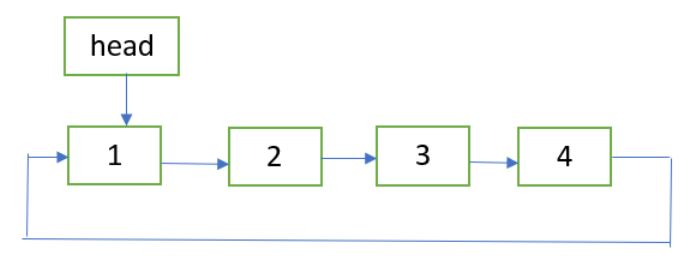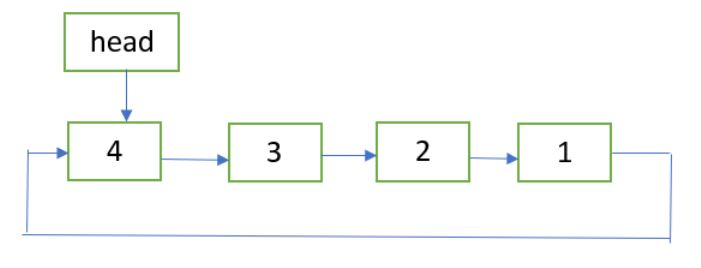GeeksforGeeks App
Open AppBrowser
Continue

# Reverse a circular linked list

Given a circular linked list of size n. The problem is to reverse the given circular linked list by changing links between the nodes.
Examples:

INPUT:OUTPUT:Approach: The approach is same as followed in reversing a singly linked list. Only here we have to make one more adjustment by linking the last node of the reversed list to the first node.

Implementation:

## C++

 `// C++ implementation to reverse``// a circular linked list``#include ` `using` `namespace` `std;` `// Linked list node``struct` `Node {``    ``int` `data;``    ``Node* next;``};` `// function to get a new node``Node* getNode(``int` `data)``{``    ``// allocate memory for node``    ``Node* newNode = ``new` `Node;` `    ``// put in the data``    ``newNode->data = data;``    ``newNode->next = NULL;``    ``return` `newNode;``}` `// Function to reverse the circular linked list``void` `reverse(Node** head_ref)``{``    ``// if list is empty``    ``if` `(*head_ref == NULL)``        ``return``;` `    ``// reverse procedure same as reversing a``    ``// singly linked list``    ``Node* prev = NULL;``    ``Node* current = *head_ref;``    ``Node* next;``    ``do` `{``        ``next = current->next;``        ``current->next = prev;``        ``prev = current;``        ``current = next;``    ``} ``while` `(current != (*head_ref));` `    ``// adjusting the links so as to make the``    ``// last node point to the first node``    ``(*head_ref)->next = prev;``    ``*head_ref = prev;``}` `// Function to print circular linked list``void` `printList(Node* head)``{``    ``if` `(head == NULL)``        ``return``;` `    ``Node* temp = head;``    ``do` `{``        ``cout << temp->data << ``" "``;``        ``temp = temp->next;``    ``} ``while` `(temp != head);``}` `// Driver program to test above``int` `main()``{``    ``// Create a circular linked list``    ``// 1->2->3->4->1``    ``Node* head = getNode(1);``    ``head->next = getNode(2);``    ``head->next->next = getNode(3);``    ``head->next->next->next = getNode(4);``    ``head->next->next->next->next = head;` `    ``cout << ``"Given circular linked list: "``;``    ``printList(head);` `    ``reverse(&head);` `    ``cout << ``"\nReversed circular linked list: "``;``    ``printList(head);` `    ``return` `0;``}`

## Java

 `// Java implementation to reverse``// a circular linked list``class` `GFG {` `    ``// Linked list node``    ``static` `class` `Node {``        ``int` `data;``        ``Node next;``    ``};` `    ``// function to get a new node``    ``static` `Node getNode(``int` `data)``    ``{``        ``// allocate memory for node``        ``Node newNode = ``new` `Node();` `        ``// put in the data``        ``newNode.data = data;``        ``newNode.next = ``null``;``        ``return` `newNode;``    ``}` `    ``// Function to reverse the circular linked list``    ``static` `Node reverse(Node head_ref)``    ``{``        ``// if list is empty``        ``if` `(head_ref == ``null``)``            ``return` `null``;` `        ``// reverse procedure same as reversing a``        ``// singly linked list``        ``Node prev = ``null``;``        ``Node current = head_ref;``        ``Node next;``        ``do` `{``            ``next = current.next;``            ``current.next = prev;``            ``prev = current;``            ``current = next;``        ``} ``while` `(current != (head_ref));` `        ``// adjusting the links so as to make the``        ``// last node point to the first node``        ``(head_ref).next = prev;``        ``head_ref = prev;``        ``return` `head_ref;``    ``}` `    ``// Function to print circular linked list``    ``static` `void` `printList(Node head)``    ``{``        ``if` `(head == ``null``)``            ``return``;` `        ``Node temp = head;``        ``do` `{``            ``System.out.print(temp.data + ``" "``);``            ``temp = temp.next;``        ``} ``while` `(temp != head);``    ``}` `    ``// Driver code``    ``public` `static` `void` `main(String args[])``    ``{``        ``// Create a circular linked list``        ``// 1.2.3.4.1``        ``Node head = getNode(``1``);``        ``head.next = getNode(``2``);``        ``head.next.next = getNode(``3``);``        ``head.next.next.next = getNode(``4``);``        ``head.next.next.next.next = head;` `        ``System.out.print(``"Given circular linked list: "``);``        ``printList(head);` `        ``head = reverse(head);` `        ``System.out.print(``            ``"\nReversed circular linked list: "``);``        ``printList(head);``    ``}``}` `// This code is contributed by Arnab Kundu`

## Python3

 `# Python3 implementation to reverse``# a circular linked list``import` `math` `# Linked list node`  `class` `Node:``    ``def` `__init__(``self``, data):``        ``self``.data ``=` `data``        ``self``.``next` `=` `None` `# function to get a new node`  `def` `getNode(data):` `    ``# allocate memory for node``    ``newNode ``=` `Node(data)` `    ``# put in the data``    ``newNode.data ``=` `data``    ``newNode.``next` `=` `None``    ``return` `newNode` `# Function to reverse the``# circular linked list`  `def` `reverse(head_ref):` `    ``# if list is empty``    ``if` `(head_ref ``=``=` `None``):``        ``return` `None` `    ``# reverse procedure same as``    ``# reversing a singly linked list``    ``prev ``=` `None``    ``current ``=` `head_ref` `    ``next` `=` `current.``next``    ``current.``next` `=` `prev``    ``prev ``=` `current``    ``current ``=` `next``    ``while` `(current !``=` `head_ref):``        ``next` `=` `current.``next``        ``current.``next` `=` `prev``        ``prev ``=` `current``        ``current ``=` `next` `    ``# adjusting the links so as to make the``    ``# last node point to the first node``    ``head_ref.``next` `=` `prev``    ``head_ref ``=` `prev``    ``return` `head_ref` `# Function to print circular linked list`  `def` `printList(head):``    ``if` `(head ``=``=` `None``):``        ``return` `    ``temp ``=` `head` `    ``print``(temp.data, end``=``" "``)``    ``temp ``=` `temp.``next``    ``while` `(temp !``=` `head):``        ``print``(temp.data, end``=``" "``)``        ``temp ``=` `temp.``next`  `# Driver Code``if` `__name__ ``=``=` `'__main__'``:` `    ``# Create a circular linked list``    ``# 1.2.3.4.1``    ``head ``=` `getNode(``1``)``    ``head.``next` `=` `getNode(``2``)``    ``head.``next``.``next` `=` `getNode(``3``)``    ``head.``next``.``next``.``next` `=` `getNode(``4``)``    ``head.``next``.``next``.``next``.``next` `=` `head` `    ``print``(``"Given circular linked list: "``,``          ``end``=``"")``    ``printList(head)` `    ``head ``=` `reverse(head)` `    ``print``(``"\nReversed circular linked list: "``,``          ``end``=``"")``    ``printList(head)` `# This code is contributed by Srathore`

## C#

 `// C# implementation to reverse``// a circular linked list``using` `System;` `class` `GFG {` `    ``// Linked list node``    ``public` `class` `Node {``        ``public` `int` `data;``        ``public` `Node next;``    ``};` `    ``// function to get a new node``    ``static` `Node getNode(``int` `data)``    ``{``        ``// allocate memory for node``        ``Node newNode = ``new` `Node();` `        ``// put in the data``        ``newNode.data = data;``        ``newNode.next = ``null``;``        ``return` `newNode;``    ``}` `    ``// Function to reverse the circular linked list``    ``static` `Node reverse(Node head_ref)``    ``{``        ``// if list is empty``        ``if` `(head_ref == ``null``)``            ``return` `null``;` `        ``// reverse procedure same as reversing a``        ``// singly linked list``        ``Node prev = ``null``;``        ``Node current = head_ref;``        ``Node next;``        ``do` `{``            ``next = current.next;``            ``current.next = prev;``            ``prev = current;``            ``current = next;``        ``} ``while` `(current != (head_ref));` `        ``// adjusting the links so as to make the``        ``// last node point to the first node``        ``(head_ref).next = prev;``        ``head_ref = prev;``        ``return` `head_ref;``    ``}` `    ``// Function to print circular linked list``    ``static` `void` `printList(Node head)``    ``{``        ``if` `(head == ``null``)``            ``return``;` `        ``Node temp = head;``        ``do` `{``            ``Console.Write(temp.data + ``" "``);``            ``temp = temp.next;``        ``} ``while` `(temp != head);``    ``}` `    ``// Driver code``    ``public` `static` `void` `Main(String[] args)``    ``{``        ``// Create a circular linked list``        ``// 1.2.3.4.1``        ``Node head = getNode(1);``        ``head.next = getNode(2);``        ``head.next.next = getNode(3);``        ``head.next.next.next = getNode(4);``        ``head.next.next.next.next = head;` `        ``Console.Write(``"Given circular linked list: "``);``        ``printList(head);` `        ``head = reverse(head);` `        ``Console.Write(``"\nReversed circular linked list: "``);``        ``printList(head);``    ``}``}` `// This code contributed by Rajput-Ji`

## Javascript

 ``

Output

```Given circular linked list: 1 2 3 4
Reversed circular linked list: 4 3 2 1 ```

Time Complexity: O(n)
Auxiliary Space: O(1)

This article is contributed by Ayush Jauhari. If you like GeeksforGeeks and would like to contribute, you can also write an article using write.geeksforgeeks.org or mail your article to review-team@geeksforgeeks.org. See your article appearing on the GeeksforGeeks main page and help other Geeks.

My Personal Notes arrow_drop_up